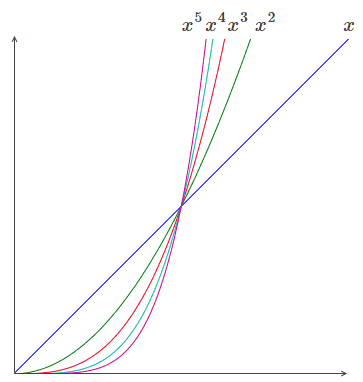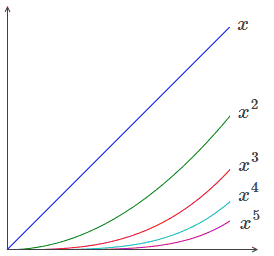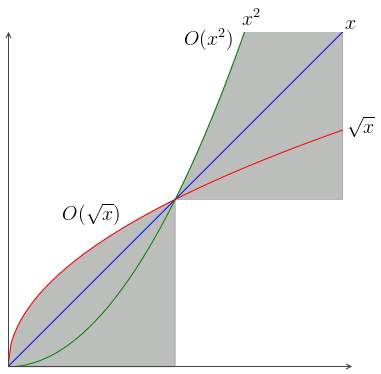# ML Wiki

## Orders of Growth

Asymptotic notation: "Big-O"

• Big-O notation gives a way to talk about the rate at which functions grow/decrease
• it allows us to study asymptotic behavior of a function

• what happens when a function becomes very very small?
• very very large?
• how fast the function approaches 0? $\infty$?

## Hierarchy of Growth/Decrease

### $x \to \infty$

Consider a Limit $\lim\limits_{x \to \infty} \cfrac{x^n}{e^x}$

• who "wins"?
• can apply L'Hopital's Rule several times:
• $\lim\limits_{x \to \infty} \cfrac{x^n}{e^x} = \lim\limits_{x \to \infty} \cfrac{n\, x^{n-1}}{e^x} = \ ... \ = \lim\limits_{x \to \infty} \cfrac{n!}{e^x}$
• $n!$ is a constant (even though it might be big - but it's still a constant)
• so $e^x$ grows faster than any Monomial Function (and any Polynomial Function as well)

We have the following hierarchy of growth:

Let's illustrate it on some monomials:

•• so we see that higher order monomials grow faster than lower order monomials

Example:

• $\lim\limits_{x \to \infty}\cfrac{3\, x^2 - 2\, x + 1}{6\, x^2 - 5\, x + 4} = \lim\limits_{x \to \infty}\cfrac{x^2\, (3 + \text{smth small})}{x^2\, (6 + \text{smth small})} = \cfrac{3}{6} = \cfrac{1}{2}$

Factorial Growth beats Exponential Growth

• let's show why:
• $\cfrac{x!}{e^x} = \cfrac{x\, (x - 1)\ ... \ 3\, 2\, 1}{e\, e \ ... \ e\, e\, e}$
• almost all terms at the numerator are greater than $e$, only 2 and 1 are smaller

### $x \to 0$

It's the opposite for $x \to 0$

• let's consider $x < 1$:
•• linear function is biggest here!
• so as $x \to 0$, $x^{n+1} < x^n$ - opposite of $x \to \infty$

The hierarchy reverses

Example:

• $\lim\limits_{x \to 0} \cfrac{1 -2x + 3x^2}{4 - 5x + 6x^2} = \cfrac{1}{4}$
• lowest order terms dominate any higher order terms

### Summary

• $x \to \infty$: high order terms dominate
• $x \to 0$: low order terms dominate

## Big-O Notation

This gives a language for describing the growth

• it has two forms
• one for $x \to \infty$
• another for $x \to 0$

Definition:

• A Function $f$ is in $O(x^n)$ if $|f(x)| < C\, |x^n|$ for some constant $C$
• more generally: $f \in O \big( g(x) \big)$ if $|f(x)| < C\, |g(x)|$ for some $C$

Interpretation:

• we care only about the upper border: "how bad can it be?"

$x \to 0$$x \to \infty$
$O(x^n)$ consists of all functions $f$ that approach 0 at least as fast as $x^n$ $O(x^n)$ consists of all functions $f$ that approach \infty no faster than $x^n$
• $\sqrt x \in O(\sqrt x)$
• $x \in O(\sqrt x)$
• $x^2 \in O(\sqrt x)$
• $\sqrt x \in O(x^2)$
• $x \in O(x^2)$
• $x^2 \in O(x^2)$

$\arctan x = x - O(x^3) = x - \cfrac{x^3}{3} + O(x^5)$

$x\, \sqrt{x^2 + 3\, x + 5} = x^2 + O(x) = x^2 + \cfrac{3}{2}\, x + O(1)$## Examples

### Case $x \to 0$

• $3\, x^2 + 5\, x \in O(x)$, but $\not \in O(x^2)$
• $\sin x \in O(x)$, $\not \in O(x^2)$
• $\ln (1 - x) - x \in O(x^2)$, $\not \in O(x^3)$
• $1 - \cos x^2 \in O(x^4)$, $\not \in O(x^5)$
• $\sqrt{n} \not \in O(x^n) \ \forall n \geqslant 1$ - it goes to zero faster than any other polynomial
• $e^{- 1/x^2} \in O(x^n) \ \forall n$

### Case $x \to \infty$

• $\arctan x \in O(1), O(n) \ \forall n \geqslant 1$
• $x\, \sqrt{1 + x^2} \in O(x^2), \ \not \in O(x^{3/2})$
• $\ln (\sinh x) \in O(x), \ \not \in O(\ln x)$: $\ln (\sinh x)$ grows too fast for $\ln x$
• $\cosh x \in O(e^x), \ \not \in O(x^n) \forall n \geqslant 0$
• $\ln x^5 \in O(\ln x), O(x^n) \ \forall n$

## Rules of Asymptotic Analysis

• $O(x^m) \cdot O(x^n) = O(x^{m + n})$
• $O(x^m) + O(x^n) = O(x^{\min m, n})$ for $x \to 0$
• $O(x^m) + O(x^n) = O(x^{\max m, n})$ for $x \to \infty$

### Examples

Example 1:

• $\cfrac{e^x}{1 - x}$ as $x \to 0$
• $\cfrac{e^x}{1 - x} = e^x\, \cfrac{1}{1-x}$
• have an exponent and a Geometric Series, can Taylor Expand both:
• $e^x\, \cfrac{1}{1-x} = \big(1 + x + O(x^2)\big) \cdot \big(1 + x + O(x^2)\big) = \ ...$
• $... \ = (1 + x)^2 + 2\, (1 + x)\, O(x) + \big( O(x^2)\big)^2 = 1 + 2x + O(x^2) + O(x^3) + O(x^4) = 1 + 2x + O(x^2)$

## Applications

• Algorithms: Computational Complexity, Big O
• Error Analysis
• Stirling Formula (see Factorial)